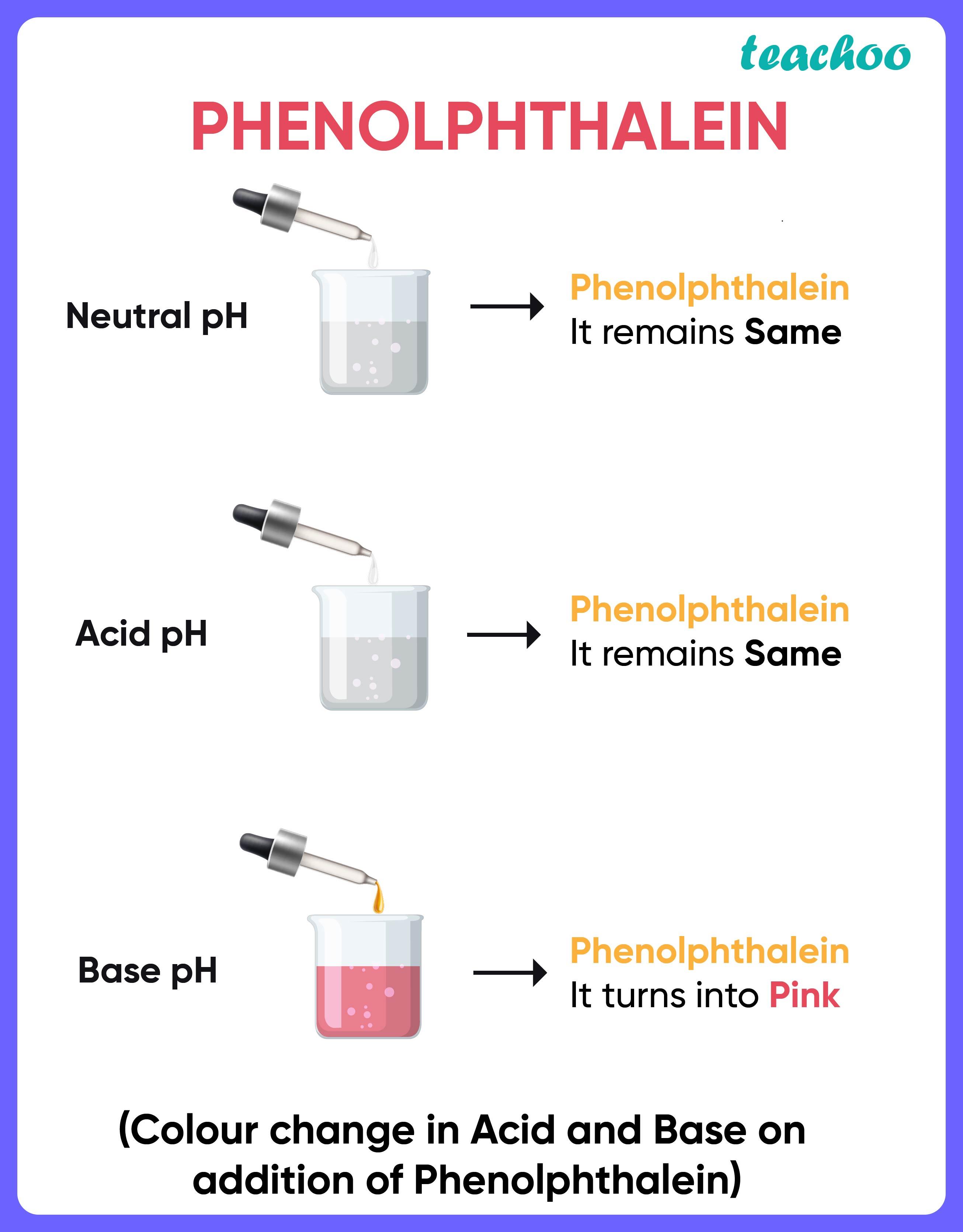Case Based Questions (MCQ)

Class 10
Chapter 2 Class 10 - Acids, Bases and Salts

## P, Q, R are different colourless solids, while S is a colourless solution. They are (in random order) Sodium  chloride (NaCl), Calcium Carbonate (CaCO 3 ), Acetic acid (CH 3 COOH) and Phenolphthalein indicator. Small  amount of the above substances were added in pairs (e.g. P with Q; Q with R etc.) to a small amount of water  in a test tube. They give the following results as shown in the observation table.  Observation Table:

 P Q R Q No Reaction − No Reaction R Dark Pink Colour No Reaction − S No Reaction No Reaction Effervescence

(a) The chemicals are:

 P Q R S (i) NaCl CaCO 3 CH 3 COOH Phenolphthalein (ii) Phenolphthalein NaCl CaCO 3 CH 3 COOH (iii) CH 3 COOH Phenolphthalein NaCl CaCO 3 (iv) CaCO 3 CH 3 COOH Phenolphthalein NaCl

Ans.Here, given compounds are

• Phenolphthalein: It is an indicator which is colorless in acidic solutions and pink in basic solutions.
• CH 3 COOH: it is an acid
• NaCl: It is a neutral salt
• CaCO 3 : it is a basic salt

Now, according to questions

• Since P only shows a color change i.e. become dark pink in color with R, this means the P is phenolphthalein and R is CaCO 3 (as it is a basic salt )
• S gives an effervescence with R. Since R is a basic salt i.e. CaCO 3 , it will react with an acid to give CO 2 which produces effervescence.
So, S is CH 3 COOH
• Thus, only NaCl remains. So, Q is NaCl

So, the correct answer is (ii)

## (iv) CaCO 3 (R) + 2CH 3 COOH (S) → (CH 3 COO)2Ca + CO 2 (effervescence) + 2H 2 O

Ans.

1. Phenolphthalein (P) + NaCl (Q) → No reaction
Since Nacl is a neutral salt, it will not give any color change with phenolphthalein.
So, this reaction is correct .

2. Phenolphthalein + CaCO 3 (R) → Alkaline medium (Dark Pink Colour)
Since CaCO3 is basic in nature, it will change the color of phenolphthalein from colorless to dark pink.
So, this reaction is correct.

3. Phenolphthalein + NaCl →Acidic medium (Blue colour)
Since NaCl is neutral, it will not show any color change with phenolphthalein.
Therefore, this reaction is incorrect.

4. CaCO 3 (R) + 2CH 3 COOH (S) → (CH 3 COO)2Ca + CO 2 (effervescence) + 2H 2 O
CaCO 3 is basic in nature and CH 3 COOH is acidic in nature. So, they both will react with each other to form salt and water and CO 2 will be released.
Hence, this reaction is correct .

So, the correct answer is (ii)

## (c) The chemicals that can be used as an acid– base indicator by a visually impaired student is  ___________.  (Petunia leaves/ Vanilla essence).

Ans.

For a visually impaired student, we need an acid base indicator whose smell changes when mixed with acid or base.

Since the smell of vanilla essence changes on mixing with acids or base.

Thus, the correct answer is Vanilla essence

Note : Vanilla essence is an olfactory indicator

## (iv) more of CH 3 COO - ions.

Ans.

An Acid is a substance which produces H + ions when dissolved in water.

• Strong acid produces more H + ions
• Weak acid produces less H + ions

Since HCl is a stronger acid, it produces more of H + ions

So, the correct answer is (iv)

Learn in your speed, with individual attention - Teachoo Maths 1-on-1 Class